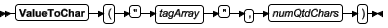# ValueToChar

This function converts an integer array of Unicode character codes to a string.

Function Group Execution Windows Embedded Thin Client Mobile Access
ValueToChar String Synchronous Supported Supported Supported Not supported

## SyntaxtagArray
The name of the integer array containing the values to be converted. If no array index is specified, then the default is 0.
Note: The tag name should be enclosed in quotes, as shown in the syntax diagram, or else the function will try to use the value of the tag.
numQtdChars
The number of values to be converted (minimum of 1), starting with the specifed array index.

## Returned value

Returns a string comprising the converted values.

## Examples

If Array = 65, Array = 66, and Array = 67, then the returned value will be “ABC”:
`  ValueToChar( "Array", 3 )  `
If Array = 65, Array = 66, and Array = 67, then the returned value will be “ABC”:
`  ValueToChar( "Array", 3 )  `